1. ### How do I solve this differential equation?

(x cos(y) + x2 +y ) dx = - (x + y2 - (x2)/2 sin y ) dy I integrated both sides 1/2x2cos(y) + 1/3 x3+xy = -xy - 1/3y3+x2cos(y) Then I get x3 + 6xy + y3 = 0 Am I doing the calculations correctly? Do I need to solve it in another way?
2. ### I Prerequisite mathematics for intermediate mechanics?

I will be taking intermediate mechanics next semester, and am a bit concerned about potential gaps in my mathematical knowledge. Long story short, I used to be a physics major, switched to electrical engineering, and then decided to double major after a semester in EE. The issue is that, as a...
3. ### Lotka Volterra estimate parameters from experimental data

Namely, in the system, I have obtained the value of parameters L, M, A and D, because I treat the other organism as equal to zero, i.e., it doesn't exist, but I am struggling about the values of B and C, that are coupled with the product of x and y. Can anyone help me how to obtain those values...
4. ### A Solving a differential equation with two unknowns

One thing that is given in paper (attached) is a operating set point for temperature which is given as 20 for day and 16 for night but I do not know whether its initial condition for temperature or not. Can anyone please guide me that what kind of equation is it and how can I solve it with these...
5. ### I The Trapping Region of the Lorenz equations

I was dealing with nonlinear systems of differential equations like the Lorenz equations (https://en.wikipedia.org/wiki/Lorenz_system). Now there is a trapping region of this system defined by the ellipsoid ρx^2+σy^2+σ(z-2ρ)^2<R. I wondered how this region is found and I found out that a...
6. ### Calculus Textbook for differentials

Hi, I am an undergrad looking to purchase a good textbook on differentials for my course which I will be taking soon, and the textbook listed for the differentials course is this one (https://www.amazon.com/gp/product/1118531779/?tag=pfamazon01-20) which apparently is not very good. So can...
7.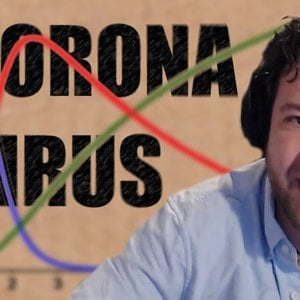### The Coronavirus Curve Simulation - Numberphile

Ben Sparks uses https://www.GeoGebra.org to explain (and code) the so-called SIR Model being used to predict the spread of cornavirus (COVID-19). A pretty cool way to visualize a set of differential equations.
8. ### How to prove this statement about the derivative of a function

My try: ##\begin{align} \dfrac{d {r^2}}{d r} \dfrac{\partial r}{\partial p} = \dfrac{\partial {r^2}}{\partial p} \tag1\\ \dfrac{\partial r}{\partial p} = \dfrac{\partial {r^2}}{\partial p} \dfrac{1}{\dfrac{d r^2}{d r}}=\dfrac{p-a\cos\theta}{r} \tag2\\ \end{align}## By chain rule...
9. ### Find the range of y in a DE

From integration by parts, and using y(10) = 0, I get the equation ##2e^{3t-30} = \frac{|y-2|}{|y+1|}.## Let ##f(t) = 2e^{3t-30}##. Since it's for t>10, f(10) = 2, and we have ##2=\frac{|y-2|}{|y+1|}##. Depending on the sign I choose to use, I get either that y=-4 or y =0. Since ##t: 10...
10. ### I E^mx and x^m

I know how to solve ODEs using both methods. The problem I'm having is knowing when to use one and not the other. If someone could help clarify this for me. I can't find the correct section in my textbook.
11. ### Differential equation problem: Modeling the spread of a rumor on campus

So this is what I have done: ##f'(t)=k*f(t)*(A-f(t))*(1-sin(\frac{pi*x}{12}))## ##\frac{1}{f(t)*(A-f(t))}=k*(1-sin(\frac{pi*x}{12}))## I see that the left can be written as this (using partial fractions): ##1/A(\frac{1}{f(t)}-\frac{1}{A-f(t)})## And then I take the integral on both sides and...
12. ### Particle Dynamics Problem (kinematics)

Summary: Mechanics problem related with Calculus (differential equations) Hi everyone, I would like some help in that task, if anyone would be willing to help :) Namely I have a problem from particle dynamics. "D:" means given info... so, D: m,g,h,b, miu. We're looking for v0 and S as given...

23. ### I Linearity of DE's

Hey all, I don't understand what makes a differential equation (DE) linear. I found this: "x y' = 1 is non-linear because y' is not multiplied by a constant" but then also this: "x' + (t^2)x = 0 is linear in x". t^2 also isn't a constant. So why is this equation linear?
24.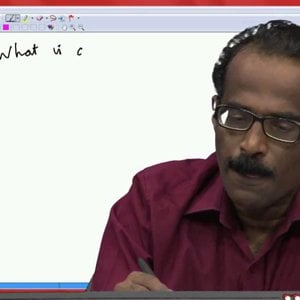### Differential Equations and Applications (NPTEL):- Lecture 01: General Introduction

COPYRIGHT strictly reserved to A. K. Nandakumaran, P. S. Datti & Raju K. George, Department of Mathematics, IISc Bangalore. Duplication PROHIBITED. Lectures: http://www.nptel.ac.in/courses/111108081/ Syllabus: http://www.nptel.ac.in/syllabus/syllabus.php?subjectId=111108081
25.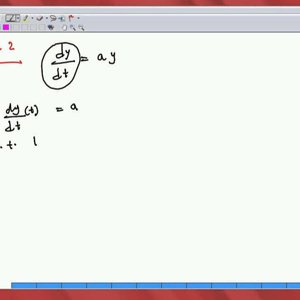### Differential Equations and Applications (NPTEL):- Lecture 02: Examples I

COPYRIGHT strictly reserved to A. K. Nandakumaran, P. S. Datti & Raju K. George, Department of Mathematics, IISc Bangalore. Duplication PROHIBITED. Lectures: http://www.nptel.ac.in/courses/111108081/ Syllabus: http://www.nptel.ac.in/syllabus/syllabus.php?subjectId=111108081
26.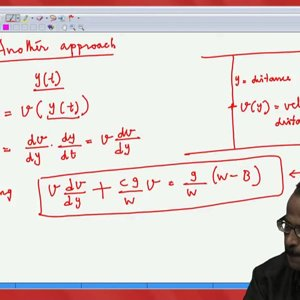### Differential Equations and Applications (NPTEL):- Lecture 03: Examples II

COPYRIGHT strictly reserved to A. K. Nandakumaran, P. S. Datti & Raju K. George, Department of Mathematics, IISc Bangalore. Duplication PROHIBITED. Lectures: http://www.nptel.ac.in/courses/111108081/ Syllabus: http://www.nptel.ac.in/syllabus/syllabus.php?subjectId=111108081
27.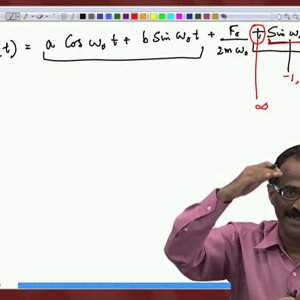### Differential Equations and Applications (NPTEL):- Lecture 04: Examples III

COPYRIGHT strictly reserved to A. K. Nandakumaran, P. S. Datti & Raju K. George, Department of Mathematics, IISc Bangalore. Duplication PROHIBITED. Lectures: http://www.nptel.ac.in/courses/111108081/ Syllabus: http://www.nptel.ac.in/syllabus/syllabus.php?subjectId=111108081
28.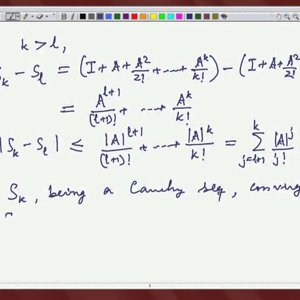### Differential Equations and Applications (NPTEL):- Lecture 05: Linear Algebra I

COPYRIGHT strictly reserved to A. K. Nandakumaran, P. S. Datti & Raju K. George, Department of Mathematics, IISc Bangalore. Duplication PROHIBITED. Lectures: http://www.nptel.ac.in/courses/111108081/ Syllabus: http://www.nptel.ac.in/syllabus/syllabus.php?subjectId=111108081
29.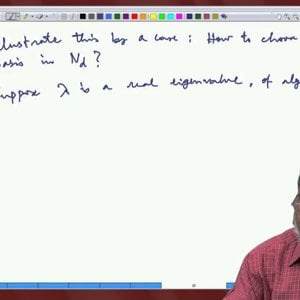### Differential Equations and Applications (NPTEL):- Lecture 06: Linear Algebra II

COPYRIGHT strictly reserved to A. K. Nandakumaran, P. S. Datti & Raju K. George, Department of Mathematics, IISc Bangalore. Duplication PROHIBITED. Lectures: http://www.nptel.ac.in/courses/111108081/ Syllabus: http://www.nptel.ac.in/syllabus/syllabus.php?subjectId=111108081
30.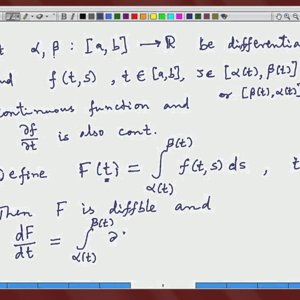### Differential Equations and Applications (NPTEL):- Lecture 07: Linear Algebra III

COPYRIGHT strictly reserved to A. K. Nandakumaran, P. S. Datti & Raju K. George, Department of Mathematics, IISc Bangalore. Duplication PROHIBITED. Lectures: http://www.nptel.ac.in/courses/111108081/ Syllabus: http://www.nptel.ac.in/syllabus/syllabus.php?subjectId=111108081